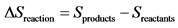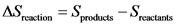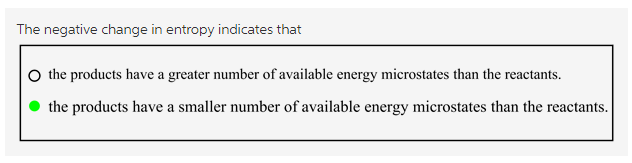# A. the products have a greater number of available energy microstates than the reactants

A negative change in entropy indicates that…

A. the products have a greater number of available energy microstates than the reactants.
B. the products have a smaller number of available energy microstates than the reactants.

Concepts and reason
The degree of randomness is termed as entropy (S). More the randomness more will be the entropy.

Fundamentals
The change in entropy for a given reaction is calculated using the formula shown below:If the summation of entropies of products are more than reactants, the entropy is positive whereas if the summation of entropies of reactants are more than products, the entropy is negative.
Boltzmann also explained entropy as a degree of the possible number of microstates for a system.